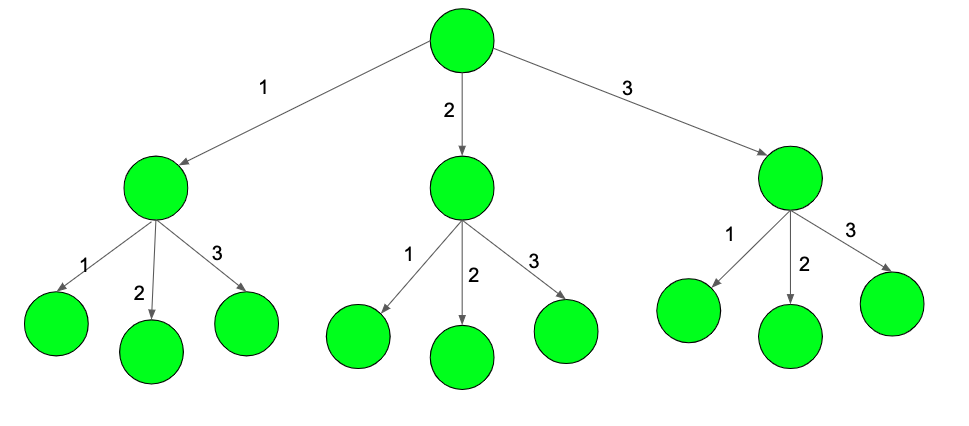Open In App

# Count number of paths whose weight is exactly X and has at-least one edge of weight M

Given an infinite tree and three numbers N, M, and X which has exactly N child from every node. Every edge has a weight of 1, 2, 3, 4..N. The task is to find the count of paths whose weight is exactly X and has a minimum of one edge of weight M in it.The diagram above shows a tree shown till level-3 and N = 3.

Examples:

```Input: N = 3, M = 2, X = 3
Output: 2
The path 1-2 and 2-1 in the image above

Input:  N = 2, M = 1, X = 4
Output:  4 ```

Approach: The problem can be solved using Dynamic Programming and memoization. We will use a top-down approach to solve this problem. Recur starting from the root with the sum initially as X, and recursively traverse all paths possible( which is from 1 to N). If the node is equal to M, then the second parameter becomes true, else it stays the same which has been passed in the previous call. Store the value in a DP[][] table to avoid visiting the same states twice.

Below is the implementation of the above approach.

## C++

 `// C++ program to count the number of paths``#include ``using` `namespace` `std;``#define max 4``#define c 2` `// Function to find the number of paths``int` `countPaths(``int` `sum, ``int` `get, ``int` `m, ``int` `n, ``int` `dp[])``{` `    ``// If the summation is more than X``    ``if` `(sum < 0)``        ``return` `0;` `    ``// If exactly X weights have reached``    ``if` `(sum == 0)``        ``return` `get;` `    ``// Already visited``    ``if` `(dp[sum][get] != -1)``        ``return` `dp[sum][get];` `    ``// Count paths``    ``int` `res = 0;` `    ``// Traverse in all paths``    ``for` `(``int` `i = 1; i <= n; i++) {` `        ``// If the edge weight is M``        ``if` `(i == m)``            ``res += countPaths(sum - i, 1, m, n, dp);``        ``else` `// Edge's weight is not M``            ``res += countPaths(sum - i, get, m, n, dp);``    ``}` `    ``dp[sum][get] = res;` `    ``return` `dp[sum][get];``}` `// Driver Code``int` `main()``{``    ``int` `n = 3, m = 2, x = 3;` `    ``int` `dp[max + 1];` `    ``// Initialized the DP array with -1``    ``for` `(``int` `i = 0; i <= max; i++)``        ``for` `(``int` `j = 0; j < 2; j++)``            ``dp[i][j] = -1;` `    ``// Function to count paths``    ``cout << countPaths(x, 0, m, n, dp);``}`

## Java

 `// Java program to count the number of paths` `public` `class` `GFG{` `    ``static` `int` `max = ``4` `;``    ``static` `int`  `c = ``2` `;``    ` `    ``// Function to find the number of paths``    ``static` `int` `countPaths(``int` `sum, ``int` `get, ``int` `m, ``int` `n, ``int` `dp[][])``    ``{``    ` `        ``// If the summation is more than X``        ``if` `(sum < ``0``)``            ``return` `0``;``    ` `        ``// If exactly X weights have reached``        ``if` `(sum == ``0``)``            ``return` `get;``    ` `        ``// Already visited``        ``if` `(dp[sum][get] != -``1``)``            ``return` `dp[sum][get];``    ` `        ``// Count paths``        ``int` `res = ``0``;``    ` `        ``// Traverse in all paths``        ``for` `(``int` `i = ``1``; i <= n; i++) {``    ` `            ``// If the edge weight is M``            ``if` `(i == m)``                ``res += countPaths(sum - i, ``1``, m, n, dp);``            ``else` `// Edge's weight is not M``                ``res += countPaths(sum - i, get, m, n, dp);``        ``}``    ` `        ``dp[sum][get] = res;``    ` `        ``return` `dp[sum][get];``    ``}``    ` `    ``// Driver Code``    ``public` `static` `void` `main(String []args)``    ``{``        ``int` `n = ``3``, m = ``2``, x = ``3``;``    ` `        ``int` `dp[][] = ``new` `int``[max + ``1``][``2``];``    ` `        ``// Initialized the DP array with -1``        ``for` `(``int` `i = ``0``; i <= max; i++)``            ``for` `(``int` `j = ``0``; j < ``2``; j++)``                ``dp[i][j] = -``1``;``    ` `        ``// Function to count paths``        ``System.out.println(countPaths(x, ``0``, m, n, dp));``    ``}``    ``// This code is contributed by Ryuga``}`

## Python3

 `# Python3 program to count the number of paths``Max` `=` `4``c ``=` `2` `# Function to find the number of paths``def` `countPaths(``Sum``, get, m, n, dp):` `    ``# If the Summation is more than X``    ``if` `(``Sum` `< ``0``):``        ``return` `0` `    ``# If exactly X weights have reached``    ``if` `(``Sum` `=``=` `0``):``        ``return` `get` `    ``# Already visited``    ``if` `(dp[``Sum``][get] !``=` `-``1``):``        ``return` `dp[``Sum``][get]` `    ``# Count paths``    ``res ``=` `0` `    ``# Traverse in all paths``    ``for` `i ``in` `range``(``1``, n ``+` `1``):` `        ``# If the edge weight is M``        ``if` `(i ``=``=` `m):``            ``res ``+``=` `countPaths(``Sum` `-` `i, ``1``, m, n, dp)``        ``else``: ``# Edge's weight is not M``            ``res ``+``=` `countPaths(``Sum` `-` `i, get, m, n, dp)``    ` `    ``dp[``Sum``][get] ``=` `res` `    ``return` `dp[``Sum``][get]` `# Driver Code``n ``=` `3``m ``=` `2``x ``=` `3``dp ``=` `[[``-``1` `for` `i ``in` `range``(``2``)]``          ``for` `i ``in` `range``(``Max` `+` `1``)]` `# Initialized the DP array with -1``for` `i ``in` `range``(``Max` `+` `1``):``    ``for` `j ``in` `range``(``2``):``        ``dp[i][j] ``=` `-``1` `# Function to count paths``print``(countPaths(x, ``0``, m, n, dp))` `# This code is contributed by Mohit kumar 29`

## C#

 `// C# program to count the number of paths``using` `System;` `class` `GFG``{``    ``static` `int` `max = 4 ;``    ``static` `int` `c = 2 ;``    ` `    ``// Function to find the number of paths``    ``static` `int` `countPaths(``int` `sum, ``int` `get``, ``int` `m,``                          ``int` `n, ``int``[, ] dp)``    ``{``    ` `        ``// If the summation is more than X``        ``if` `(sum < 0)``            ``return` `0;``    ` `        ``// If exactly X weights have reached``        ``if` `(sum == 0)``            ``return` `get``;``    ` `        ``// Already visited``        ``if` `(dp[sum, ``get``] != -1)``            ``return` `dp[sum, ``get``];``    ` `        ``// Count paths``        ``int` `res = 0;``    ` `        ``// Traverse in all paths``        ``for` `(``int` `i = 1; i <= n; i++)``        ``{``    ` `            ``// If the edge weight is M``            ``if` `(i == m)``                ``res += countPaths(sum - i, 1, m, n, dp);``            ``else` `// Edge's weight is not M``                ``res += countPaths(sum - i, ``get``, m, n, dp);``        ``}``    ` `        ``dp[sum, ``get``] = res;``    ` `        ``return` `dp[sum, ``get``];``    ``}``    ` `    ``// Driver Code``    ``public` `static` `void` `Main()``    ``{``        ``int` `n = 3, m = 2, x = 3;``    ` `        ``int``[,] dp = ``new` `int``[max + 1, 2];``    ` `        ``// Initialized the DP array with -1``        ``for` `(``int` `i = 0; i <= max; i++)``            ``for` `(``int` `j = 0; j < 2; j++)``                ``dp[i, j] = -1;``    ` `        ``// Function to count paths``        ``Console.WriteLine(countPaths(x, 0, m, n, dp));``    ``}``}` `// This code is contributed by Akanksha Rai`

## PHP

 ``

## Javascript

 ``

Output

`2`

Complexity Analysis:

• Time Complexity: O(x*n), as we are using a loop to traverse n times and in each traversal, we are recursively calling the function again which will cost O(x). Where n is the number of children from every node and x is the total weight.
• Auxiliary Space: O(x*n), as we are using extra space for the DP matrix. Where n is the number of children from every node and x is the total weight.